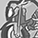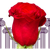# QlikView App Dev

Discussion Board for collaboration related to QlikView App Development.

cancel
Showing results for
Did you mean:Contributor

## Rolling 12 Month Bar Chart with Set Analysis

Hello,

Trying to get a Bar chart of the last rolling year's EBITDA and cannot get it to work ( I would still consider myself as a rookie)

My Dimension is: %MonthYear in the following format "Jan 2020".

Expression that I am trying to use this board to come up at the moment:

Sum({<%MonthYear ={">=\$(=Date(AddMonths(Max(%MonthYear), -11), 'MMM YYYY')<=\$(=Date(Max(%MonthYear), 'MMM YYYY'))"},Year=, Month=>}\$(vActEBITDA))

Variable for vActEBITDA:

RangeSum(
Sum({\$<RecordTypeDesc={'Actual'},GLAccountTreeNm4={'1153\Net income (loss)'}>}Amount),
Sum({\$<RecordTypeDesc={'Actual'},GLAccountTreeNm6={'1884\Interest expense'}>}Amount),
Sum({\$<RecordTypeDesc={'Actual'},GLAccountTreeNm7={'1607\Depreciation & Amortization'}>}Amount))

Let me know if you need actual data to help with this and I can throw together an example app.

1 Solution

Accepted SolutionsPartner - Master III

let's try this:

sum(
aggr(
rangesum(
above(
Sum( {\$< RecordTypeDesc = {'Actual'}, GLAccountTreeNm4 = {'1153\Net income (loss)'} >} Amount )
+
Sum( {\$< RecordTypeDesc = {'Actual'}, GLAccountTreeNm6 = {'1884\Interest expense'} >} Amount )
+
Sum( {\$< RecordTypeDesc = {'Actual'}, GLAccountTreeNm7 = {'1607\Depreciation & Amortization'} >} Amount )
,
0, 12 ) ), %MonthYear ) )

5 RepliesPartner - Master III

maybe this:

Sum( {\$< %MonthYear = {">=\$(=Date(AddMonths(Max(%MonthYear), -11), 'MMM YYYY')<=\$(=Date(Max(%MonthYear), 'MMM YYYY'))"}, RecordTypeDesc = {'Actual'}, GLAccountTreeNm4 = {'1153\Net income (loss)'} >} Amount )
+
Sum( {\$< %MonthYear = {">=\$(=Date(AddMonths(Max(%MonthYear), -11), 'MMM YYYY')<=\$(=Date(Max(%MonthYear), 'MMM YYYY'))"}, RecordTypeDesc = {'Actual'}, GLAccountTreeNm6 = {'1884\Interest expense'} >} Amount )
+
Sum( {\$< %MonthYear = {">=\$(=Date(AddMonths(Max(%MonthYear), -11), 'MMM YYYY')<=\$(=Date(Max(%MonthYear), 'MMM YYYY'))"}, RecordTypeDesc = {'Actual'}, GLAccountTreeNm7 = {'1607\Depreciation & Amortization'} >} Amount )Contributor
Author

Hmmm, I tried that and got the "No Data to display" errorCreator

Did you try a rangesum with total?

93/93Partner - Master III

let's try this:

sum(
aggr(
rangesum(
above(
Sum( {\$< RecordTypeDesc = {'Actual'}, GLAccountTreeNm4 = {'1153\Net income (loss)'} >} Amount )
+
Sum( {\$< RecordTypeDesc = {'Actual'}, GLAccountTreeNm6 = {'1884\Interest expense'} >} Amount )
+
Sum( {\$< RecordTypeDesc = {'Actual'}, GLAccountTreeNm7 = {'1607\Depreciation & Amortization'} >} Amount )
,
0, 12 ) ), %MonthYear ) )Contributor
Author

That did it!  Amazing Thank you!